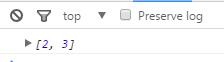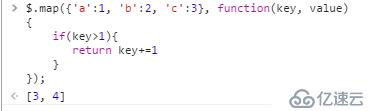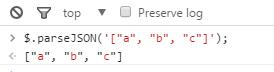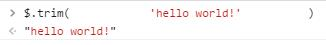# Zepto.js 核心方法

\$( )

1、\$( htmlString ) 创建元素

```//创建元素
var p1 =\$('<p>Hello Zepto</p>');

\$('body').append(p1);```

2、\$( htmlString, attributes ) 创建带有属性的元素

```//创建带有属性的元素
var p2 =\$('<p />',{text:'Hello World!', id:'greeting', css:{color:'red', fontSize:'30px', fontWeight:'bold'}});
\$(p2).appendTo('body');```3、Zepto(function(\$){ ... } )  DOM加载完毕，调用这个方法

```//当页面ready的时候，执行回调
Zepto(function(\$){
})```\$.camelCase( string )     => string\$.contains(parent, node)    => boolean

\$.each(collection, function(index, item){ ... })   =>collection

（1）、遍历数组（2）、遍历对象\$.extend(target, [source, [source2, ...] ])   => target

\$.extend(true, target, [source, ...])             => target

```var target = {one: 'targetObject'};
var source = {two: 'sourceObject'};
\$.extend(target, source);
console.log(target);```\$.fn

Zepto.fn 是一个对象，它拥有 Zepto 对象上所有可用的方法，如 addClass( ), attr( ) 和其他方法。在这个对象添加一个方法，所有的 Zepto 对象上都能用到该方法。

```\$.fn.empty = function(){
return this.each(function(){
this.innerHTML == '';
})
}```

\$.grep(items, function(item){ ... } )   => array

```var arr = \$.grep([1,2,3], function(item){
return item>1
});
console.log( arr );```\$.inArray(element, array, [fromIndex] )   => number

[fromIndex]参数：可选，表示从哪个索引值开始向后查找\$.isArray( object )   => boolean\$.isFunction( object )   => booleand

\$.isPlainObject( object )   => boolean\$.isWindow( object )   => boolean

\$.map(collection, function(item, index){ ... })   => collection\$.parseJSON( string )   => object\$.trim( string )   => string\$.type( object )   => string